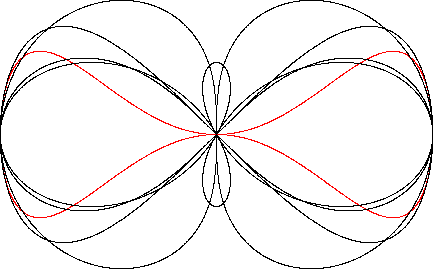Dumbbell Curve
DUMBBELL CURVE

Balmoral Software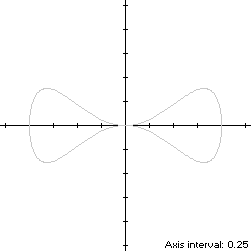The dumbbell curve is a bisymmetric sextic curve S with parametric equations
x(t) = cos(t)

y(t) = cos2(t)sin(t), 0 ≤ t < 2π

The curve follows a counterclockwise path around the origin, starting from its right edge at (1,0). Its extreme abscissa points are (±1,0) and its maximum ordinate point in the first quadrant is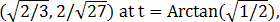so the width x height of its bounding rectangle is### Metrics

The perimeter of S is 5.541003 and its area is π/4.

### Convex Hull

The convex hull is created by connecting the extreme ordinate points with two horizontal line segments of length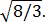We have
x'(t) = -sin(t)

y'(t) = 3cos3(t) - 2cos(t),

so by (L1), the perimeter of the convex hull is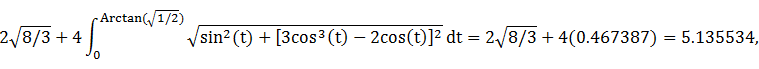which is about 7% shorter than the perimeter of the dumbbell curve.

The line segments of the convex hull create a rectangle of area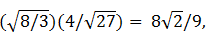as shown in blue in the left diagram below. By (A1), the area of the convex hull is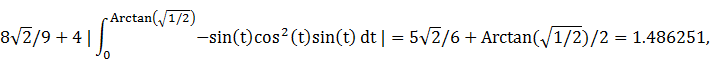which is almost twice the area of the dumbbell curve.

### Circumcircle

The maximum squared distance
x2(t) + y2(t) = cos2(t) + cos4(t)sin2(t)
is 1, so that is the circumradius.

### Circumellipse

From Lemma B, we have
x(t)y(t) = cos3(t)sin(t)
This expression is maximized in the first quadrant at t* = π/6, so the circumellipse dimensions are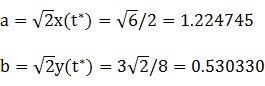For verification, we have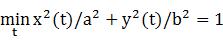### Incircle (lobe)

Consider the right lobe of the dumbbell curve, where -π/2 ≤ t < π/2. The maximum ordinate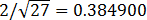of the lobe does not define its inradius since the corresponding abscissa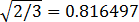is too close to the right edge (1,0), so its incircle is constrained by the right edge of S. Using z = 1 in Lemma C,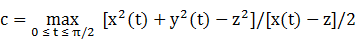is an extremely complicated expression approximated by 0.704642. The corresponding radius R = |c - z| = 0.295358. For verification, we have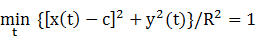### Inellipse (lobe)

Using z = 1 in Lemma E,
d/dt [x(t) - z]y(t) = d/dt [cos(t) - 1]cos2(t)sin(t)
has a zero at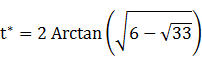The corresponding coordinates are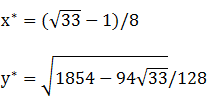We then have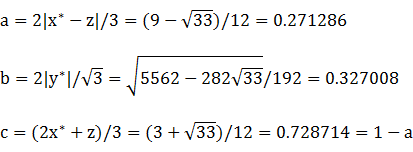For verification, we have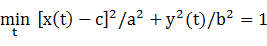### Summary Table

 Perimeter Area Centroid Figure Parameters Incircle (lobe) R = 0.295358 1.855791 0.274062 (0.704642,0) Inellipse (lobe)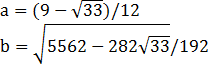1.883674 0.278699 (0.728714,0) Dumbbell curve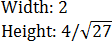5.541003 0.785398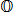Convex hull 5.135534 1.885618 Circumellipse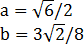5.731721 2.040524 Circumcircle R = 1 6.283185 3.141593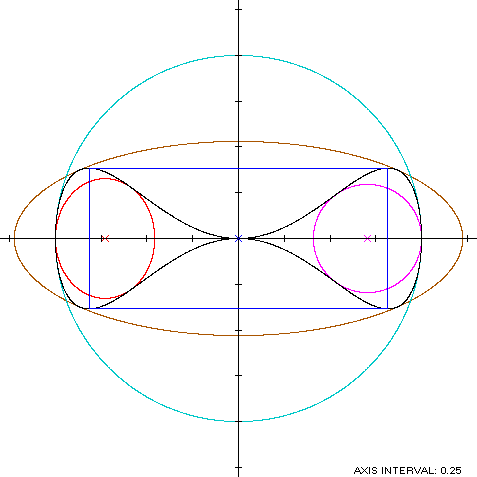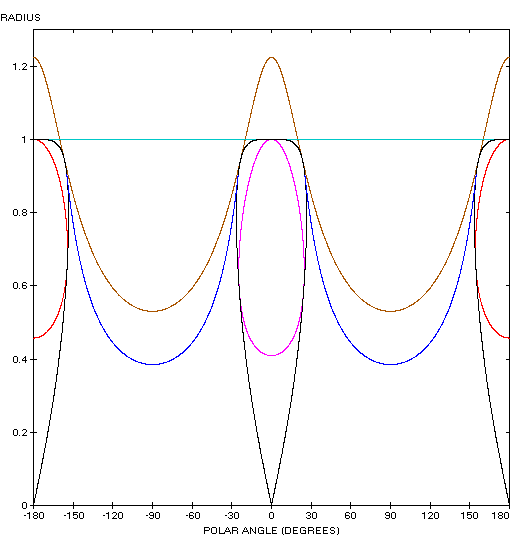Peripheral Vision

The dumbbell curve (red) is a member of a group of figure-8 curves described on these pages, including (inside to outside) the bowtie, the Lemniscate of Bernoulli, the Lemniscate of Gerono and the dipole: